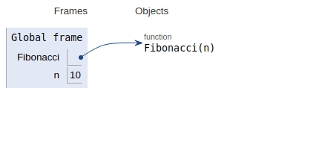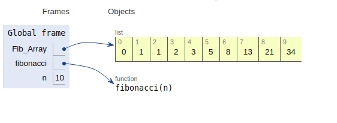# N-th Fibonacci number in Python Program

A fibbonacci number is defined by the recurrance relation given below:

Fn = Fn-1 + Fn-2

With F0 = 0 and F1 = 1.

First few fibbonacci numbers are 0,1,1,2,3,5,8,13,..................

We can compute the Fibonacci numbers using the method of recursion and dynamic programming.

Now let’s see the implementation in the form of Python script

## Example

Live Demo

#recursive approach
def Fibonacci(n):
if n<0:
print("Fibbonacci can't be computed")
# First Fibonacci number
elif n==1:
return 0
# Second Fibonacci number
elif n==2:
return 1
else:
return Fibonacci(n-1)+Fibonacci(n-2)
# main
n=10
print(Fibonacci(n))

## Output

34

The scope of all the variables declared is shown below## Example

Live Demo

#dynamic approach
Fib_Array = [0,1]
def fibonacci(n):
if n<0:
print("Fibbonacci can't be computed")
elif n<=len(Fib_Array):
return Fib_Array[n-1]
else:
temp = fibonacci(n-1)+fibonacci(n-2)
Fib_Array.append(temp)
return temp
# Driver Program
n=10
print(fibonacci(n))

## Output

34

The scope of all the variables declared is shown below## Conclusion

In this article, we learned about computation of nth Fibonacci number using recursion and dynamic programming approach.